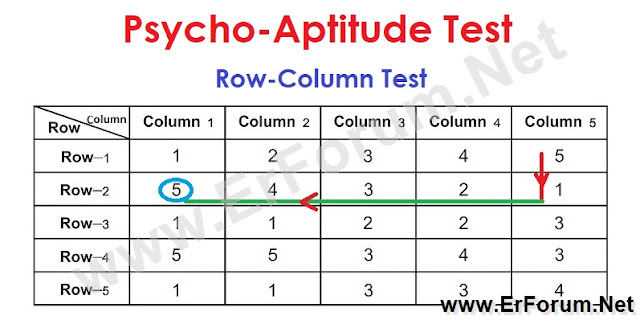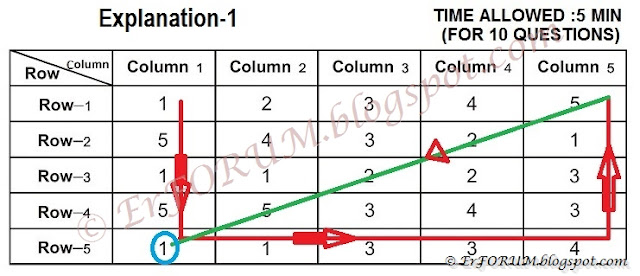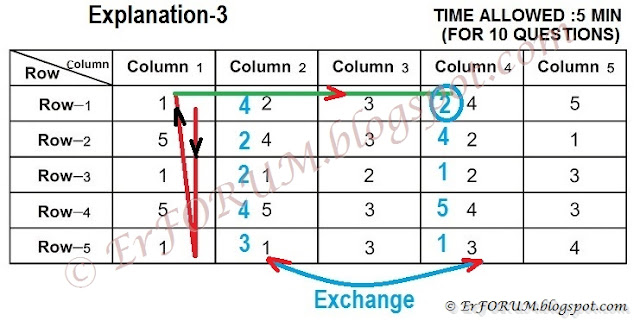3/recent/ticker-posts

# Table Test or Row-Column Test of Railway Psycho Exam

## Table Test or Row-Column Test of Railway Psycho Exam

### Test of Measuring Ability to Follow Directions

Following Direction Test include two types of tests-
1. Table Test/Row-Column Test
2. Clock Test/Watch-Table Test

Table Test
This test comes under “Test-2” (Following direction test) of ALP Psycho.
As a table constitutes of Row and Column so this test is also called a Column-Row Test.
In this category of test, 10 questions are asked based on a given table with an allowable time of 5 minutes.

Before start solving questions based on Row-column method (Table test) we have to understand the few terms of this test.#### Basic concepts of Table test

This test is asked with showing a table followed by 10 questions.

You have to solve all those 10 questions based on the given table.
What is a Table?
A table consists of a number of rows and columns.

#### What are Rows and Columns?

Rows are horizontal and columns are vertical lines.

See the below table to understand row and column.
 Row /Column Column 1 Column 2 Column 3 Column 4 Column 5 Row 1 D C B C C Row 2 A A C E D Row 3 B E D E B Row 4 D C B B A Row 5 E D A C A

Understanding of row and columns are very essential to solving questions based on the table test. So let us do a few questions:
Q1. Which letter is present in the 5th row and 3rd column?
A. – “A”
Q2. Which letter is present in the 3rd row and 3rd column?
A. – “D”
Q3. Which letter is present in the 4th row and 3rd column?
A. – “B”
Q4. Which letter is present in the 1st row and 5th column?
A. – “C”
Q5. Which letter is present in the 3rd row and 4th column?
A. – “E”
Now we assume that you become perfect in understanding Row and Column of any given table.

#### Diagonal of a Table

What is diagonal? ( विकर्ण किसे कहते  हैं ? )
दो सम्मुख शीर्षो को मिलाने वाले सरल रेखा-खण्ड को विकर्ण (डायगोनल) कहते हैं।

There are 4 diagonal of a table
Diagonal of 1st row (Pankti 1 ka vikarn)
Diagonal of the 1st column (Stambh 1 ka vikarn)
Diagonal of 5th row (Pankti 5 ka vikarn)
Diagonal of 5th column (Stambh 5 ka Vikarn)

After understanding these basics of Table test lets start solving questions.
However, a few other terms are also important but we learn those terms at the time explanation of the questions.

See Few Example below:-Table Test (Concept+Practice Sets)@Rs50
Key Features:
Solved Examples and Practice sets
110+ Practice Question with Answer Key
Medium- Bilingual (English+Hindi)

1.